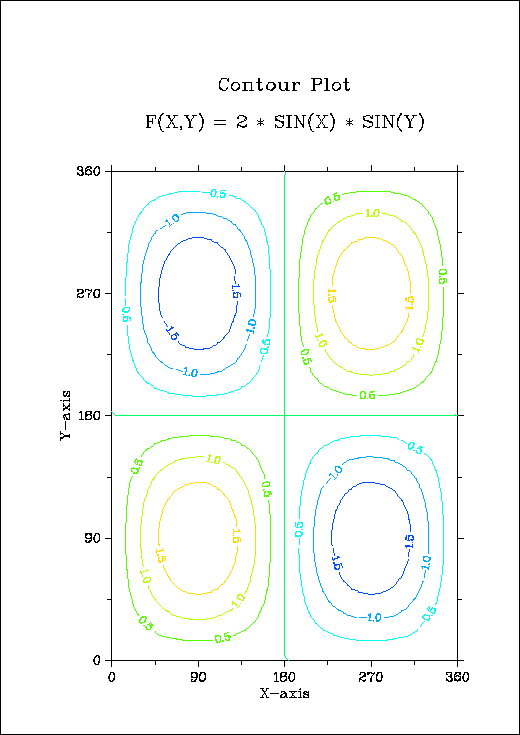## B.4 Contour Plot

```%GCL
// Contour Plot
N = 100
FLOAT ZMAT[N,N], XRAY[N]

FPI = 3.1415927 / 180.
STEP = 360. / (N - 1)
X = FALLOC (N) * STEP
Y = FALLOC (N) * STEP

DO J = 0, N - 1
ZMAT[*,J] = 2 * SIN (X * FPI) * SIN (Y[J] * FPI)
END DO

METAFL ('CONS')
SETPAG ('DA4P')
DISINI ()
COMPLX ()
PAGERA ()

INTAX  ()
AXSPOS (450, 2670)

NAME   ('X-axis', 'X')
NAME   ('Y-axis', 'Y')
TITLIN ('Contour plot', 2)
TITLIN ('F(X,Y) = 2 * SIN(X) * SIN(Y)', 4)

GRAF (0.,360.,0.,90.,0.,360.,0.,90.)

HEIGHT (30)
DO I = 1,9
ZLEV = -2.0 + (I - 1) * 0.5
IF (I == 5)
LABELS ('NONE', 'CONTUR')
ELSE
LABELS ('FLOAT', 'CONTUR')
END IF
CONTUR (X, N, Y, N, ZMAT, ZLEV)
END DO

HEIGHT (50)
TITLE  ()
DISFIN ()
```Figure B.4: Contour Plot

Next | Previous | Contents